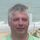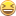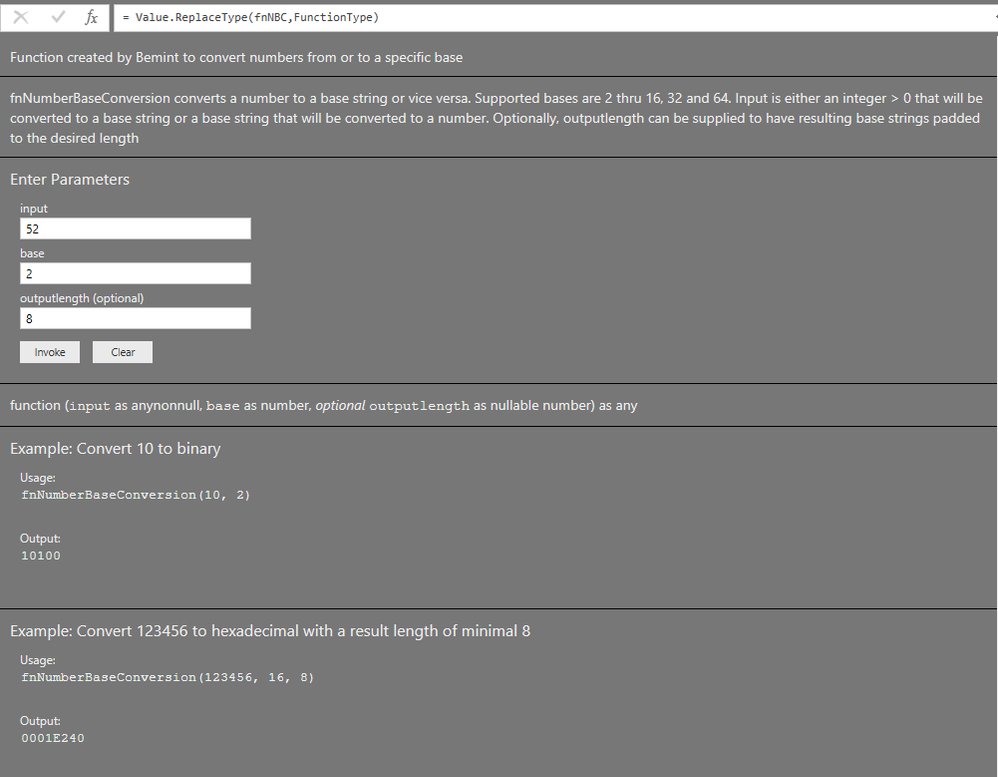cancel
Showing results for
Did you mean:Frequent Visitor

## Number to Binary

Is it possible to convert numbers to binary data in Power BI?

1 ACCEPTED SOLUTIONCommunity Champion

I addition to my previous respons.

Some of the things I realized recently, is that:

an "if ... then ... else"  statement with big chunks of code like above, is not required.

Thanks to concept of "Lazy Evaluation" in Power Query, you can just put in the code without if...then...else.

Intead, the choice is made later. In the example below: in the Result step.

So the inner part of the previous function (which is the actual function without documentation), can be rewritten as:

```    fnNBC = (input as anynonnull, base as number, optional outputlength as number) as any =>
let
//    input = 10,
//    base = 2,
//    outputlength = null,
Base16 = "0123456789ABCDEF",
Base32 = "ABCDEFGHIJKLMNOPQRSTUVWXYZ234567",
Base64 = "ABCDEFGHIJKLMNOPQRSTUVWXYZabcdefghijklmnopqrstuvwxyz0123456789+/",
Lookups = List.Zip({{16,32,64},{Base16,Base32,Base64}}),
Lookup = Text.ToList(List.Last(List.Select(Lookups,each _{0} <= List.Max({16, base}))){1}),
InputToList = Text.ToList(input),

// This part will be executed if input is text:
Reversed = List.Reverse(InputToList),
BaseValues = List.Transform(Reversed, each List.PositionOf(Lookup,_)),
Indexed = List.Zip({BaseValues, {0..Text.Length(input)-1}}),
Powered = List.Transform(Indexed, each _{0}*Number.Power(base,_{1})),
Decimal = List.Sum(Powered),
// So far this part

// This part will be executed if input is not text:
Elements = 1+Number.RoundDown(Number.Log(input,base),0),
Powers = List.Transform(List.Reverse({0..Elements - 1}), each Number.Power(base,_)),
ResultString = List.Accumulate(Powers,
[Remainder = input,String = ""],
(c,p) => [Remainder = c[Remainder] - p * Number.RoundDown(c[Remainder] / p,0),
String = c[String] & Lookup{Number.RoundDown(c[Remainder]/p,0)}])[String],
// So far this part

Result = if input is text then Decimal else PaddedResultString
in
Result```

Specializing in Power Query Formula Language (M)
9 REPLIES 9Super User

In Power Query you can use Binary.FromText.

http://www.excelandpowerbi.com/?p=291

Become an expert!: Enterprise DNA
External Tools: MSHGQM
Latest book!:
Mastering Power BI 2nd EditionDAX is easy, CALCULATE makes DAX hard...Frequent Visitor

Thanks for the input. I tried using the approach to convert the number '52'  to binary, but can't the expected output of 00110100. Is there another feature I'm missing to use numbers instead of hex or text?Community Champion

I don't get it. All the binary functions seem to expect a binary value or text representing a binary value as the input. I don't see any function anywhere that converts base 10 to base 2.

Edit: seems a solution was presented while I was reading M documentation.

Proud to be a Super User!Community Champion

Still a reaction to your now-striked-through-text, just for better understanding:

in Power Query, "Binary" means: the binary representation of - for example - file contents, not a binary value as in 1001 = 9.

Of course: file contents are also a series of 0's and 1's, but they typically don't represent 1 (huge) decimal value.Specializing in Power Query Formula Language (M)Community Champion

Got it. Thanks.

Proud to be a Super User!Anonymous
Not applicable

I know it is an old topic, but I just tried to reuse this code snippet, and it does not work for 0 and "0". Has anybody made that correction?Community Champion

It should work with "0", but not if the base is 32, which has no "0" in the 32 characters.

A small correction to have it work with 0:
in step "Elements" I added a List.Max formula, in order to supply a minimum of 1 as argument to Number.Log.

```fnNBC = (input as anynonnull, base as number, optional outputlength as number) as any =>
let
//    input = 10,
//    base = 2,
//    outputlength = null,
Base16 = "0123456789ABCDEF",
Base32 = "ABCDEFGHIJKLMNOPQRSTUVWXYZ234567",
Base64 = "ABCDEFGHIJKLMNOPQRSTUVWXYZabcdefghijklmnopqrstuvwxyz0123456789+/",
Lookups = List.Zip({{16,32,64},{Base16,Base32,Base64}}),
Lookup = Text.ToList(List.Last(List.Select(Lookups,each _{0} <= List.Max({16, base}))){1}),
InputToList = Text.ToList(input),

// This part will be executed if input is text:
Reversed = List.Reverse(InputToList),
BaseValues = List.Transform(Reversed, each List.PositionOf(Lookup,_)),
Indexed = List.Zip({BaseValues, {0..Text.Length(input)-1}}),
Powered = List.Transform(Indexed, each _{0}*Number.Power(base,_{1})),
Decimal = List.Sum(Powered),
// So far this part

// This part will be executed if input is not text:
Elements = 1+Number.RoundDown(Number.Log(List.Max({1,input}),base),0),
Powers = List.Transform(List.Reverse({0..Elements - 1}), each Number.Power(base,_)),
ResultString = List.Accumulate(Powers,
[Remainder = input,String = ""],
(c,p) => [Remainder = c[Remainder] - p * Number.RoundDown(c[Remainder] / p,0),
String = c[String] & Lookup{Number.RoundDown(c[Remainder]/p,0)}])[String],
// So far this part

Result = if input is text then Decimal else PaddedResultString
in
Result```

Specializing in Power Query Formula Language (M)Community Champion

Some time ago I shared a great function on another forum.

With this function - fnNumberBaseConversion - you can convert numbers to (almost) any base and vice versa.

Disclaimer: as far as I know, the function works fine, but I can't give any guarantees.

Screen shot with the parameters to get 00110100 from 52:Result:And the code, with complete documentation:

```let
Documentation =
[Documentation.Category =    "Custom Function",
Documentation.Description = "Function created by Bemint to convert numbers from or to a specific base",
Documentation.Examples =    { [Description = "Convert 10 to binary",
Code = "fnNumberBaseConversion(10, 2)",
Result = "10100"],
[Description = "Convert 123456 to hexadecimal with a result length of minimal 8",
Code = "fnNumberBaseConversion(123456, 16, 8)",
Result = "0001E240"]},
Documentation.LongDescription =
"fnNumberBaseConversion converts a number to a base string or vice versa.
Supported bases are 2 thru 16, 32 and 64.
Input is either an integer > 0 that will be converted to a base string or a base string that will be converted to a number.
Optionally, outputlength can be supplied to have resulting base strings padded to the desired length"],

fnNBC = (input as anynonnull, base as number, optional outputlength as number) as any =>
let
//    input = 10,
//    base = 2,
//    outputlength = null,
Base16 = "0123456789ABCDEF",
Base32 = "ABCDEFGHIJKLMNOPQRSTUVWXYZ234567",
Base64 = "ABCDEFGHIJKLMNOPQRSTUVWXYZabcdefghijklmnopqrstuvwxyz0123456789+/",
Lookups = List.Zip({{16,32,64},{Base16,Base32,Base64}}),
Lookup = Text.ToList(List.Last(List.Select(Lookups,each _{0} <= List.Max({16, base}))){1}),
InputToList = Text.ToList(input),
Result = if input is text
then
let
Reversed = List.Reverse(InputToList),
BaseValues = List.Transform(Reversed, each List.PositionOf(Lookup,_)),
Indexed = List.Zip({BaseValues, {0..Text.Length(input)-1}}),
Powered = List.Transform(Indexed, each _{0}*Number.Power(base,_{1})),
Decimal = List.Sum(Powered)
in
Decimal
else
let
Elements = 1+Number.RoundDown(Number.Log(input,base),0),
Powers = List.Transform(List.Reverse({0..Elements - 1}), each Number.Power(base,_)),
ResultString = List.Accumulate(Powers,
[Remainder = input,String = ""],
(c,p) => [Remainder = c[Remainder] - p * Number.RoundDown(c[Remainder] / p,0),
String = c[String] & Lookup{Number.RoundDown(c[Remainder]/p,0)}])[String],
in
in
Result,
// Create FunctionType with metadata at function type level:
FunctionType = Type.ForFunction([ReturnType = type any, Parameters = [input = type anynonnull, base = type number, outputlength = type number]], 2) meta Documentation,
fnNumberBaseConversion = Value.ReplaceType(fnNBC,FunctionType)
in
fnNumberBaseConversion```
Specializing in Power Query Formula Language (M)Community Champion

I addition to my previous respons.

Some of the things I realized recently, is that:

an "if ... then ... else"  statement with big chunks of code like above, is not required.

Thanks to concept of "Lazy Evaluation" in Power Query, you can just put in the code without if...then...else.

Intead, the choice is made later. In the example below: in the Result step.

So the inner part of the previous function (which is the actual function without documentation), can be rewritten as:

```    fnNBC = (input as anynonnull, base as number, optional outputlength as number) as any =>
let
//    input = 10,
//    base = 2,
//    outputlength = null,
Base16 = "0123456789ABCDEF",
Base32 = "ABCDEFGHIJKLMNOPQRSTUVWXYZ234567",
Base64 = "ABCDEFGHIJKLMNOPQRSTUVWXYZabcdefghijklmnopqrstuvwxyz0123456789+/",
Lookups = List.Zip({{16,32,64},{Base16,Base32,Base64}}),
Lookup = Text.ToList(List.Last(List.Select(Lookups,each _{0} <= List.Max({16, base}))){1}),
InputToList = Text.ToList(input),

// This part will be executed if input is text:
Reversed = List.Reverse(InputToList),
BaseValues = List.Transform(Reversed, each List.PositionOf(Lookup,_)),
Indexed = List.Zip({BaseValues, {0..Text.Length(input)-1}}),
Powered = List.Transform(Indexed, each _{0}*Number.Power(base,_{1})),
Decimal = List.Sum(Powered),
// So far this part

// This part will be executed if input is not text:
Elements = 1+Number.RoundDown(Number.Log(input,base),0),
Powers = List.Transform(List.Reverse({0..Elements - 1}), each Number.Power(base,_)),
ResultString = List.Accumulate(Powers,
[Remainder = input,String = ""],
(c,p) => [Remainder = c[Remainder] - p * Number.RoundDown(c[Remainder] / p,0),
String = c[String] & Lookup{Number.RoundDown(c[Remainder]/p,0)}])[String],
// So far this part

Result = if input is text then Decimal else PaddedResultString
in
Result```

Specializing in Power Query Formula Language (M)